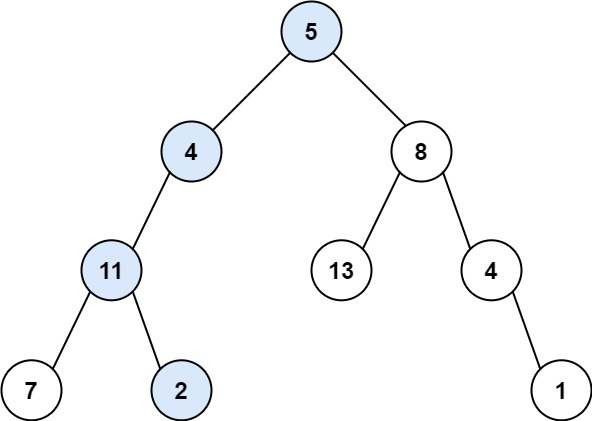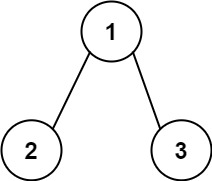Coding

# LeetCode Path Sum Solution

0
(0)

Given the `root` of a binary tree and an integer `targetSum`, return `true` if the tree has a root-to-leaf path such that adding up all the values along the path equals `targetSum`.

leaf is a node with no children.

Example 1:```Input: root = [5,4,8,11,null,13,4,7,2,null,null,null,1], targetSum = 22
Output: true
Explanation: The root-to-leaf path with the target sum is shown.
```

Example 2:```Input: root = [1,2,3], targetSum = 5
Output: false
Explanation: There two root-to-leaf paths in the tree:
(1 --> 2): The sum is 3.
(1 --> 3): The sum is 4.
There is no root-to-leaf path with sum = 5.
```

Example 3:

```Input: root = [], targetSum = 0
Output: false
Explanation: Since the tree is empty, there are no root-to-leaf paths.
```

Constraints:

• The number of nodes in the tree is in the range `[0, 5000]`.
• `-1000 <= Node.val <= 1000`
• `-1000 <= targetSum <= 1000`

Solution

What you need to find out is if there exists any path from root to leaf such that sum of those elements is equal to targetSum. As with any binary tree problem I started to try with recursion.

If there are no elements, then by default answer is false since there are no root to leaf paths.

Otherwise check if there is path in left subtree or right subtree whose sum is equal to targetSum minus value of current node. If either of these paths exists, then return true.

Below is the java code for the same.

``````public boolean hasPathSum(TreeNode root, int targetSum) {
if (root == null) return false;
else if (root.left == null && root.right == null) return root.val == targetSum;
else return hasPathSum(root.left, targetSum-root.val) || hasPathSum(root.right, targetSum-root.val);``````

And below is the efficiency. It is 100% faster than any LeetCode submitted solution. 🙂If you are able to understand why we are subtracting value of current node from targetSum while calling the function recursively on left or right subtree, then you will solve the problem easily.

Click on a star to rate it!

Average rating 0 / 5. Vote count: 0

No votes so far! Be the first to rate this post.

As you found this post useful...

Follow us on social media!

We are sorry that this post was not useful for you!

Let us improve this post!

Tell us how we can improve this post?